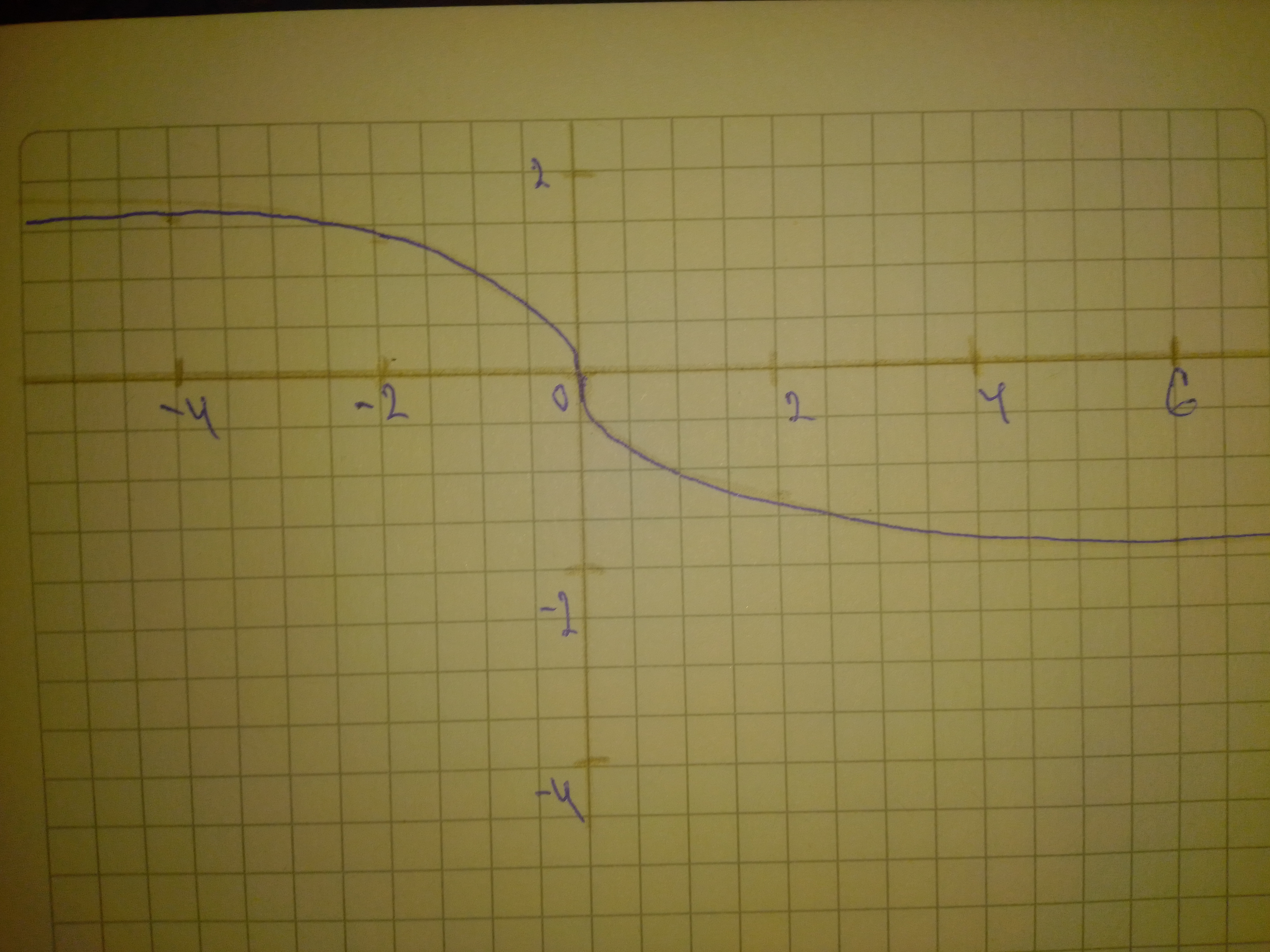Ask question

# Sketch a graph of the function. Use transformations of functions when ever possible. f(x)=sqrt{-x}# Sketch a graph of the function. Use transformations of functions when ever possible. f(x)=sqrt{-x}

Question
Transformations of functionsasked 2021-01-16
Sketch a graph of the function. Use transformations of functions when ever possible. $$\displaystyle{f{{\left({x}\right)}}}=\sqrt{{{3}}}{\left\lbrace-{x}\right\rbrace}$$

## Answers (1)2021-01-17
Step 1
Shown is the graph of $$\displaystyle{f{{\left({x}\right)}}}=\sqrt{{{3}}}{\left\lbrace-{x}\right\rbrace}$$
x is on the horizontal axis and y is on the vertical axis.
Step 2### Relevant Questionsasked 2021-05-04
For each of the following functions f (x) and g(x), express g(x) in the form a: f (x + b) + c for some values a,b and c, and hence describe a sequence of horizontal and vertical transformations which map f(x) to g(x).
$$\displaystyle{f{{\left({x}\right)}}}={x}^{{2}}+{3},{g{{\left({x}\right)}}}={x}^{{2}}-{6}{x}+{8}$$asked 2021-02-05
Sketch a graph of the function. Use transformations of functions when ever possible. $$\displaystyle{f{{\left({x}\right)}}}={1}\ -\ \sqrt{{{x}\ +\ {2}}}$$asked 2021-06-06
For each of the following functions f (x) and g(x), express g(x) in the form a: f (x + b) + c for some values a,b and c, and hence describe a sequence of horizontal and vertical transformations which map f(x) to g(x).
$$\displaystyle{f{{\left({x}\right)}}}={x}^{{2}}-{2},{g{{\left({x}\right)}}}={2}+{8}{x}-{4}{x}^{{2}}$$asked 2021-05-08
For each of the following functions f (x) and g(x), express g(x) in the form a: f (x + b) + c for some values a,b and c, and hence describe a sequence of horizontal and vertical transformations which map f(x) to g(x).
$$\displaystyle{f{{\left({x}\right)}}}={x}^{{2}},{g{{\left({x}\right)}}}={3}{x}^{{2}}-{24}{x}+{8}$$asked 2021-05-01
For each of the following functions f (x) and g(x), express g(x) in the form a: f (x + b) + c for some values a,b and c, and hence describe a sequence of horizontal and vertical transformations which map f(x) to g(x).
$$\displaystyle{f{{\left({x}\right)}}}={x}^{{2}},{g{{\left({x}\right)}}}={2}{x}^{{2}}+{4}{x}$$asked 2021-02-25
Sketch a graph of the function. Use transformations of functions when ever possible. $$\displaystyle{f{{\left({x}\right)}}}=\ {\frac{{{1}}}{{{3}}}}{\left({x}\ -\ {5}\right)},\ {2}\ \leq\ {x}\ \leq\ {8}$$asked 2021-02-03
Sketch a graph of the function. Use transformations of functions when ever possible. $$\displaystyle{f{{\left({x}\right)}}}=\ {\frac{{{1}}}{{{\left({x}\ -\ {1}\right)}^{{{3}}}}}}$$asked 2020-12-01
Sketch a graph of the function. Use transformations of functions when ever possible.
$$\displaystyle{f{{\left({x}\right)}}}={2}{x}^{{{2}}}-{1}$$asked 2020-10-20
Sketch a graph of the function. Use transformations of functions when ever possible.
$$\displaystyle{f{{\left({x}\right)}}}={\left|{x}+{1}\right|}$$asked 2021-01-02
Sketch a graph of the function. Use transformations of functions when ever possible. $$\displaystyle{f{{\left({x}\right)}}}=\ -{\frac{{{1}}}{{{x}^{{{2}}}}}}$$
...